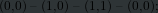# 关于在org文件中用Tikz绘图

#+OPTIONS:\usepackage{tikz}
#+begin_src latex
\begin{figure}
\begin{tikzpicture}
\draw (0,0) -- (1,0) -- (1,1) -- (0,0);
\end{tikzpicture}
\end{figure}
#+end_src
2 个赞

https://orgmode.org/manual/Exporting-code-blocks.html

#+OPTIONS:\usepackage{tikz}
#+begin_src latex  :exports results
\begin{figure}
\begin{tikzpicture}
\draw (0,0) -- (1,0) -- (1,1) -- (0,0);
\end{tikzpicture}
\end{figure}
#+end_src


#+OPTIONS:\usepackage{tikz}
#+begin_src latex  :exports results :file test.png
\begin{figure}
\begin{tikzpicture}
\draw (0,0) -- (1,0) -- (1,1) -- (0,0);
\end{tikzpicture}
\end{figure}
#+end_src#+OPTIONS 的用法不对吧，试试

#+LATEX_HEADER: \usepackage{tikz}

\begin{figure}[htbp]
\centering
\begin{tikzpicture}
\draw (0,0) -- (1,0) -- (1,1) -- (0,0);
\end{tikzpicture}
\end{figure}


https://leeifrankjaw.github.io/notes/automata/

<policy domain="coder" rights="none" pattern="PDF" />


<policy domain="coder" rights="read|write" pattern="PDF" />


https://orgmode.org/worg/org-contrib/babel/languages/ob-doc-LaTeX.html

1 个赞